1. Which of the following calculations gives the largest answer?

2. Nadiya is baking a cake. The recipe says that her cake should be baked in the oven for 1 hour and 35 minutes. She puts the cake in the oven at 11:40 am.

At what time should she take the cake out of the oven?

3. What is the value of x ?

A:
B:
C:
D:
E:

A: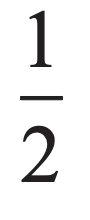B: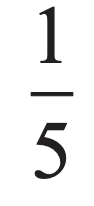C: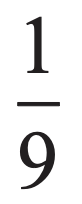D: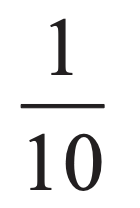E: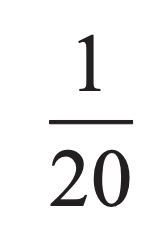5. What is the value of 201 × 7 − 7 × 102?

B:
C:
D:
E:

6. In a magic square, the numbers in each row, each column and the two main diagonals have the same total.

This magic square uses the integers 2 to 10.

Which of the following are the missing cells?

A: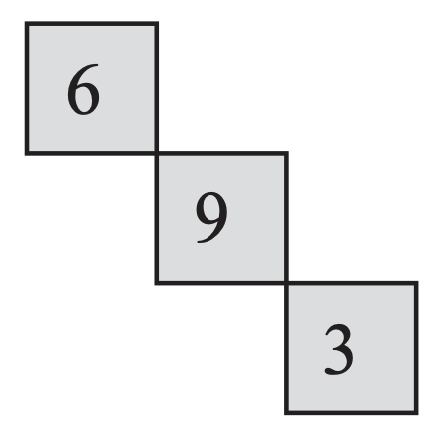B: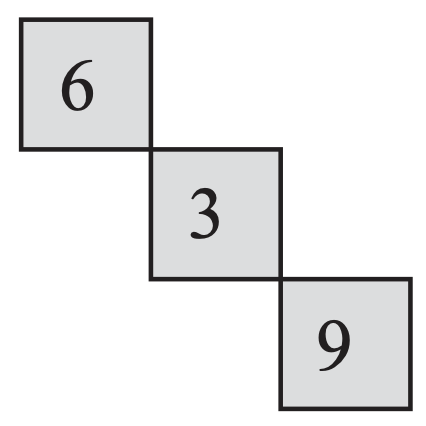C: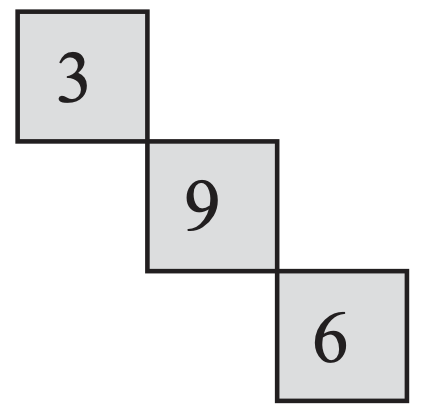D: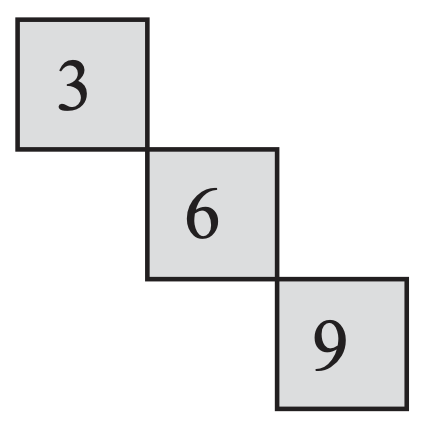E: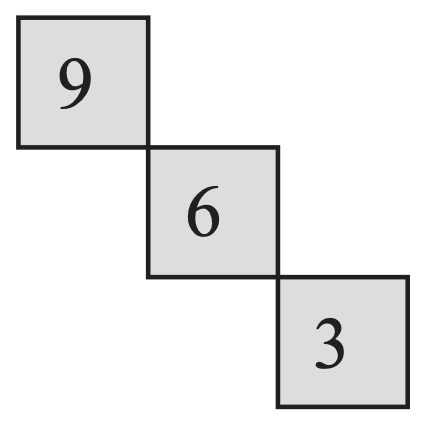7. If you work out the values of the following expressions and then place them in increasing numerical order, which comes in the middle?

A: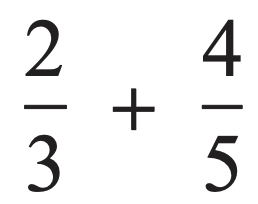B: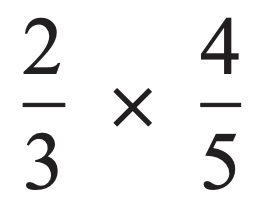C: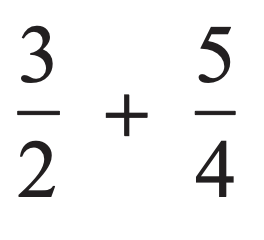D: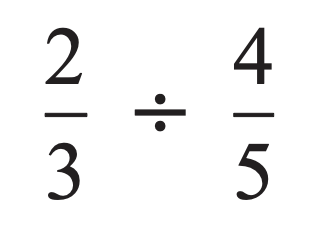E: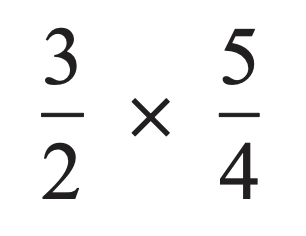8. The diagram shows a rectangle PQRS and T is a point on PS such that QT is perpendicular to RT. The length of QT is 4 cm. The length of RT is 2 cm.

What is the area of the rectangle PQRS?

A:B:C:D:E:9. In William Shakespeare's play As You Like It, Rosalind speaks to Orlando about “He that will divide a minute into a thousand parts”.

Which of the following is equal to the number of seconds in one thousandth of one minute?

A:
B:
D:

10. Which of the following integers is not a multiple of 45?

A:B:C:D:E:11. Seven squares are drawn on the sides of a heptagon so that they are outside the heptagon, as shown in the diagram.

What is the sum of the seven marked angles?

A:B:C:D:E:12. Last year, at the school where Gill teaches Mathematics, 315 out of the 600 pupils were girls. This year, the number of pupils in the school has increased to 640. The proportion of girls is the same as it was last year.

How many girls are there at the school this year?

A:B:C:D:E:13. Consider the following three statements.

(i)  Doubling a positive number always makes it larger.

(ii)  Squaring a positive number always makes it larger.

(iii)  Taking the positive square root of a positive number always makes it smaller.

Which statements are true?

A:B:C:D:E:14. Mathias is given a grid of twelve small squares. He is asked to shade grey exactly four of the small squares so that his grid has two lines of reflection symmetry.

How many different grids could he produce?

A:B:C:D:E:15. What is the remainder when the square of 49 is divided by the square root of 49?

A:B:C:D:E:Back to the top